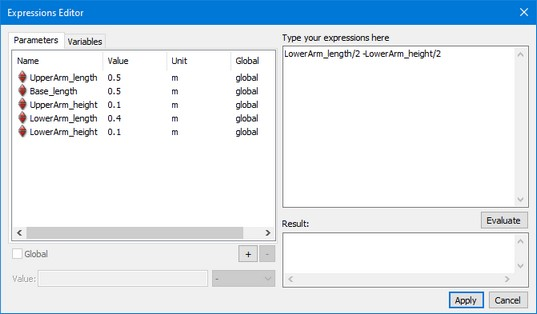﻿ 20-sim webhelp > Toolboxes > 3D Mechanics Toolbox > Parametric Models > Expressions Editor

# Expressions Editor

The Expressions Editor enables you to enter values in the 3D Mechanics Editor which are calculated from expression rather than a hard value.## Expressions Editor

You can type the expressions in the right upper part of the editor. Expressions are similar to the left hand side of an equation in the Equations Editor. Check the proper use of expressions by clicking the Evaluate button. The output or an error message will then be displayed in the Results section at the right lower part.

You can use parameters in the expressions typing by double-clicking in the parameters list at the top left of the editor. Click on the + sign to add new parameters. You can make a parameter global, by selecting the global selection.

## Expressions

The standard arithmetic functions and operators can be used in expressions:

cos(x)

sin (y)

tan(x)

x*y

y/x

2*pi

x^2

exp(y)

log(x)

sqrt(y)

if ((x > 0.1),1,2)

abs(x)

round(x)